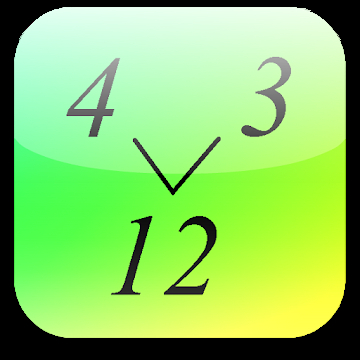#### Alphabetical

A | B | C | D | E | F | G | H | I | J | K | L | M | N | O | P | Q | R | S | T | U | V | W | X | Y | Z

# Least Common MultipleThis free math calculator lets you calculate the least common multiple of two numbers. <br><br>Best mathematical tool for school and college! If you are a student, it will helps you to learn arithmetics and calculations with numbers.